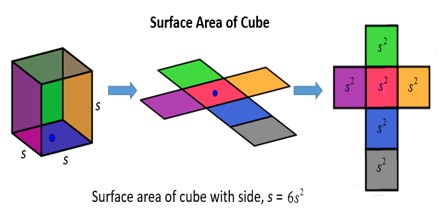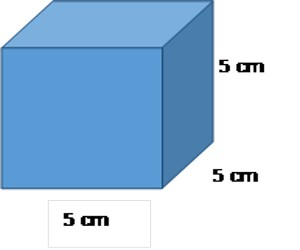In general, the surface area is the sum of all the areas of all the shapes that cover the surface of the object. To calculate the surface are of a cube, find the surface area of one side and multiply by 6. The surface area of any side is the length of a side squared.

Example: Surface area of a cube with a side of length 4 = 4x4x6 = 96Looking at the cube template, it is easy to see that the cube has six sides and each side is a square

The area of one square is a × a = a2

Since there are six sides, the total surface area, call it SA is:

SA = a2 + a2 + a2 + a2 + a2 + a2

SA = 6 × a2

In words, the surface area of a cube is the area of the six squares that cover it. The area of one of them is a*a, or a2. Since these are all the same, you can multiply one of them by six, so the surface area of a cube is 6 times one of the sides squared.

Example:Find the surface area if the length of one side is 5 cm

Surface area = 6 × a2

Surface area = 6 × 52

Surface area = 6 × 5 × 5

Surface area = 150 cm2

Units

Remember that the length of an edge and the surface area will be in similar units. So if the edge length is in miles, then the surface area will be in square miles, and so on.